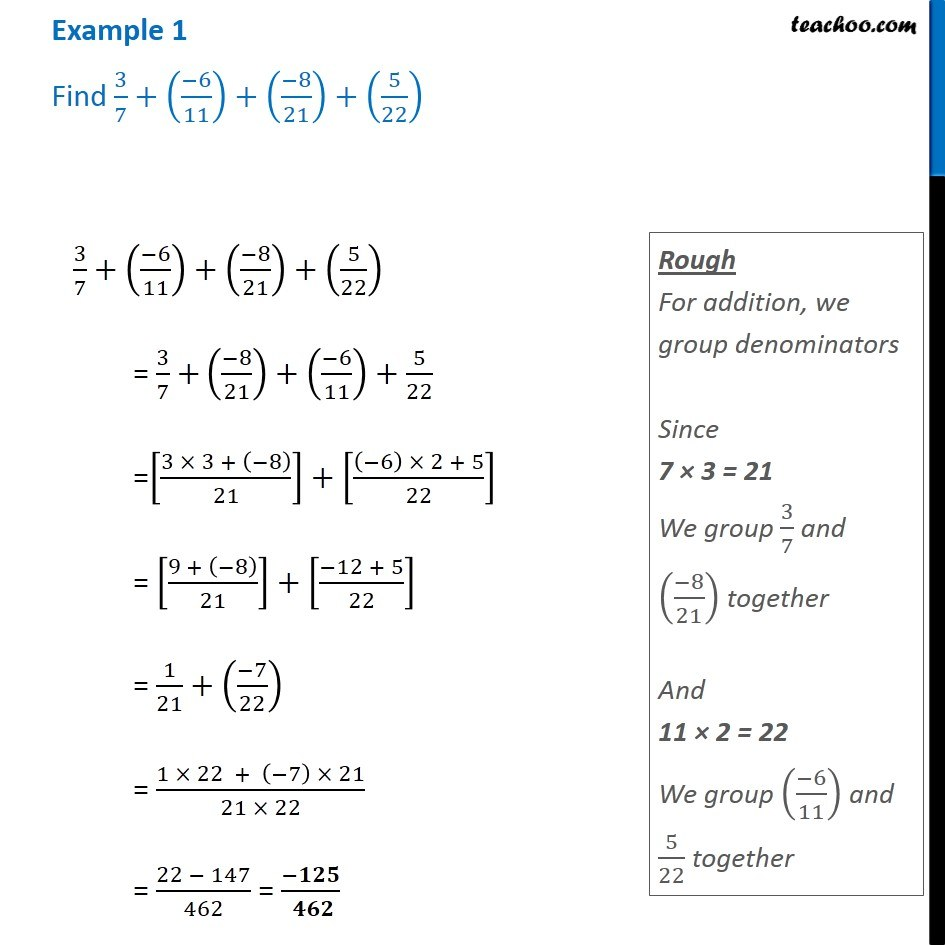Addition and Multiplication of Rational numbers

Chapter 1 Class 8 Rational Numbers
Concept wiseLearn in your speed, with individual attention - Teachoo Maths 1-on-1 Class

### Transcript

Example 1 Find 3/7+((−6)/11)+((−8)/21)+(5/22) 3/7+((−6)/11)+((−8)/21)+(5/22) = 3/7 +((−8)/21)+((−6)/11)+5/22 =[(3 × 3 + (−8))/21]+[((−6) × 2 + 5)/22] = [(9 + (−8))/21]+[(−12 + 5)/22] = 1/21+((−7)/22) = (1 × 22 + (−7) × 21)/(21 × 22) = (22 − 147)/462 = (−𝟏𝟐𝟓)/( 𝟒𝟔𝟐) Rough For addition, we group denominators Since 7 × 3 = 21 We group 3/7 and ((−8)/21) together And 11 × 2 = 22 We group ((−6)/11) and 5/22 together